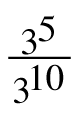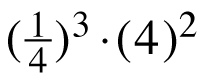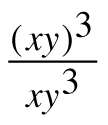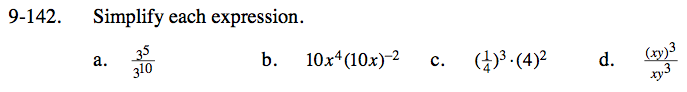Home > CC3 > Chapter 9 > Lesson 9.2.6 > Problem9-142

9-142.
1. Simplify each expression. Homework Help ✎

1.2. 10x4(10x)−2

3.4.3(5 − 10)

$3^{-5} = \frac{1}{3^{5}}$

Remember, when an exponent is located outside of a set of parentheses, the exponent applies to every term inside the parentheses.

10x4(10−2x−2)

$\frac{{\it x}^{2}}{10}$

This can be rewritten as:

(4−1)3(4)2

See (b).

See (a) and (b).